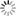# Episode List

OR

### 1962

1962
Admiral Chester NimitzKnow what this is about?
Be the first one to add a plot.

1962
Admiral Richard E. ByrdKnow what this is about?
Be the first one to add a plot.

1962
Adolf Hitler: Part 1
7.4Know what this is about?
Be the first one to add a plot.

1962
Adolf Hitler: Part 2Know what this is about?
Be the first one to add a plot.

1962
Babe RuthHe is perhaps the greatest sports hero in American history. George Herman "Babe" Ruth, Jr., was more than just a ballplayer--he was a beloved public figure whose popularity at one time seemed to transcend that of baseball itself. Profane and earthy, "The Babe" had a warmth of spirit about him that both electrified and inspired the nation. In his lifetime, he set several Major League records-the most notable of which took place in 1927 when he hit a record-breaking 60 home runs in a single season. From his early childhood in Baltimore to the infamous 1919 sale by the ...

1962
Benito MussoliniKnow what this is about?
Be the first one to add a plot.

1962
Clarence DarrowKnow what this is about?
Be the first one to add a plot.

1962
Colonel Billy MitchellKnow what this is about?
Be the first one to add a plot.

1962
Dag HammarskjoldKnow what this is about?
Be the first one to add a plot.

1962
Fiorello La GuardiaKnow what this is about?
Be the first one to add a plot.

1962
Francisco FrancoKnow what this is about?
Be the first one to add a plot.

1962
Franklin Delano Roosevelt: Part 2 - The War YearsKnow what this is about?
Be the first one to add a plot.

1962
Franklin Delano Roosevelt: Part I - The New DealKnow what this is about?
Be the first one to add a plot.

1962
General Charles De GaulleKnow what this is about?
Be the first one to add a plot.

1962
General Douglas MacArthurKnow what this is about?
Be the first one to add a plot.

1962
George PattonKnow what this is about?
Be the first one to add a plot.

1962
Harry S. Truman: Part 1Know what this is about?
Be the first one to add a plot.

1962
Harry S. Truman: Part 2Know what this is about?
Be the first one to add a plot.

1962
Henry FordKnow what this is about?
Be the first one to add a plot.

1962
John L. LewisKnow what this is about?
Be the first one to add a plot.

1962
John PershingKnow what this is about?
Be the first one to add a plot.

1962
Josef StalinKnow what this is about?
Be the first one to add a plot.

1962
Knute RockneKnow what this is about?
Be the first one to add a plot.

1962
Mahatma GandhiKnow what this is about?
Be the first one to add a plot.

1962
Pope Pius XIIKnow what this is about?
Be the first one to add a plot.

1962
Robert A. TaftKnow what this is about?
Be the first one to add a plot.

1962
Senator Joseph McCarthyKnow what this is about?
Be the first one to add a plot.

1962
The Duke of WindsorKnow what this is about?
Be the first one to add a plot.

1962
Theodore RooseveltKnow what this is about?
Be the first one to add a plot.

1962
Thomas EdisonKnow what this is about?
Be the first one to add a plot.

1962
Wernher von BraunKnow what this is about?
Be the first one to add a plot.

1962
Winston Churchill: The Darkest HoursKnow what this is about?
Be the first one to add a plot.

1962
Winston Churchill: The Final VictoryKnow what this is about?
Be the first one to add a plot.

1962
Woodrow WilsonKnow what this is about?
Be the first one to add a plot.

« 1961 |  1962  1963  »